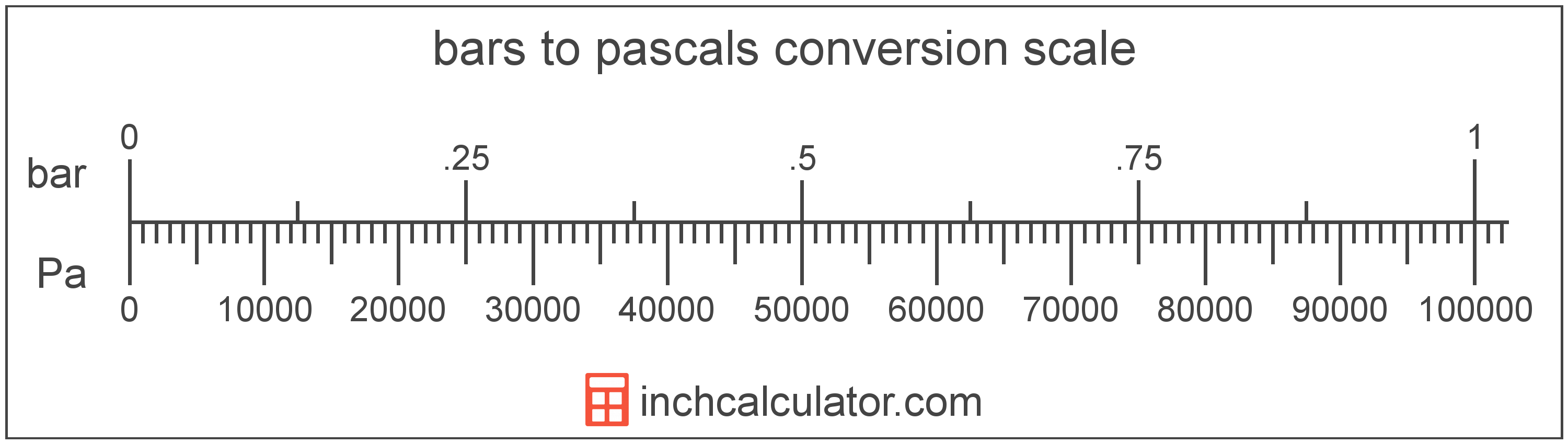# Bars to Pascals Converter

Enter the pressure in bars below to get the value converted to pascals.

Results in Pascals:1 bar = 100,000 Pa

Do you want to convert pascals to bars?

## How to Convert Bars to Pascals

To convert a measurement in bars to a measurement in pascals, multiply the pressure by the following conversion ratio: 100,000 pascals/bar.

Since one bar is equal to 100,000 pascals, you can use this simple formula to convert:

pascals = bars × 100,000

The pressure in pascals is equal to the pressure in bars multiplied by 100,000.

For example, here's how to convert 5 bars to pascals using the formula above.
pascals = (5 bar × 100,000) = 500,000 Pa## What is a Bar?

The bar is equal to 100,000 pascals, which are defined as the pressure of one newton per square meter. One bar is just less than the average atmospheric pressure.

The bar is a non-SI metric unit for pressure. Bars can be abbreviated as bar; for example, 1 bar can be written as 1 bar.

## What is a Pascal?

The pascal is defined as the pressure of one newton per square meter.

The pascal is the SI derived unit for pressure in the metric system. Pascals can be abbreviated as Pa; for example, 1 pascal can be written as 1 Pa.

Pascals can be expressed using the formula:
1 Pa = 1 N / m2

Pressure in pascals is equal to the force in newtons divided by the area in square meters.

## Bar to Pascal Conversion Table

Table showing various bar measurements converted to pascals.
Bars Pascals
0.00001 bar 1 Pa
0.00002 bar 2 Pa
0.00003 bar 3 Pa
0.00004 bar 4 Pa
0.00005 bar 5 Pa
0.00006 bar 6 Pa
0.00007 bar 7 Pa
0.00008 bar 8 Pa
0.00009 bar 9 Pa
0.000001 bar 0.1 Pa
0.00001 bar 1 Pa
0.0001 bar 10 Pa
0.001 bar 100 Pa
0.01 bar 1,000 Pa
0.1 bar 10,000 Pa
1 bar 100,000 Pa